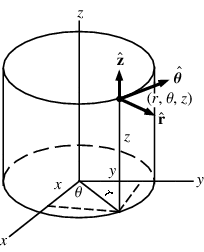# Cylindrical to rectangular coordinates

• violette

#### violette

Hi sorry,I still need some help on converting coordinates >.<

Set up an integral in rectangular coordinates equivalent to the integral

∫($0 ≤ θ ≤ \frac{∏}{2})$∫($1 ≤ r ≤ \sqrt{3})$∫(1 ≤ z ≤ √(4-r2)) r3(sinθcosθ)z2 dz dr dθ

Arrange the order of integration to be z first,then y,then x.

I manage to convert,however the answer has 2 parts and I only managed to get 1 part.How do I know if the answer will have more than 1 part?

Hi again violette!What did you convert?
What are parts 1 and 2?
Did you already try to make a drawing?

Hi I like Serena,really thanks so much for being so helpful =D

hmm...how do I draw with cylindrical coordinates?I only know how to make a drawing given rectangular coords >.<

this was what I got:
∫(0 ≤ z ≤ 1)∫(√(1-x2) ≤ y ≤ √(3-x2)∫(1 ≤ x ≤ √(4-x2-y2) z2xy dzdydx

Hi I like Serena,really thanks so much for being so helpful =D

hmm...how do I draw with cylindrical coordinates?I only know how to make a drawing given rectangular coords >.<

Well, you draw in rectangular coordinates, but you add circles for constant r.

Here's an example:this was what I got:
∫(0 ≤ z ≤ 1)∫(√(1-x2) ≤ y ≤ √(3-x2)∫(1 ≤ x ≤ √(4-x2-y2) z2xy dzdydx

But that's looking good!You only seem to have switched x and z around in the limits or something.
And the limits (0 ≤ ° ≤ 1) are not right.

How did you get that?

omg thanks so much!The diagram made it easier for me to try on my own =)

Ah oopx,it should be this:
∫(0 ≤ x ≤ 1)∫(√(1-x2) ≤ y ≤ √(3-x2)∫(1 ≤ z ≤ √(4-x2-y2) z2xy dzdydx

Hmm,actually I got 3 values for x after all the conversion: 0,1 and $\sqrt{3}$.
But I used 0 and 1 because they are the limits that fitted y

omg thanks so much!The diagram made it easier for me to try on my own =)

Ah oopx,it should be this:
∫(0 ≤ x ≤ 1)∫(√(1-x2) ≤ y ≤ √(3-x2)∫(1 ≤ z ≤ √(4-x2-y2) z2xy dzdydx

Hmm,actually I got 3 values for x after all the conversion: 0,1 and $\sqrt{3}$.
But I used 0 and 1 because they are the limits that fitted y

Yes, that's basically it.
The upper limit for x is still $\sqrt{3}$. You cannot just discard that part of the object.
However, the lower limit for y needs to be modified to 0 if it would otherwise be undefined.
In your diagram you should be able to see why that is.

You can write that for instance like √max(0, 1-x2) ≤ y ≤ √(3-x2).